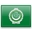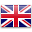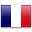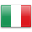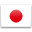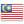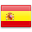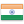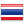VCE Specialist Mathematics - Gladstone Park

# VCE Specialist Mathematics

https://www.vcaa.vic.edu.au/curriculum/vce/vce-study-designs/specialistmathematics/Pages/Index.aspx

Is this subject available for acceleration? NO

Specialist Mathematics consists of the following areas of study: Functions and Graphs, Algebra, Calculus, Vectors, Mechanics and Probability and Statistics.

This link shows a selection of jobs that have some relation to the subject of: Specialist Mathematics

##### Units 1 and 2
• Must be taken in conjunction with Year 11 Mathematical Methods Units 1 and 2
• Designed for students who are intending to continue with VCE Specialist Mathematics Units 3 and 4

##### Units 3 and 4
• Must be taken in conjunction with Mathematical Methods Units 3 & 4

##### Skills
• Gain an understanding of natural numbers and related proofs
• Represent plane vectors as directed lines segments involving position, displacement and velocity
• Interpret graphical representation of data
• Graph simple reciprocal functions including, sine, cosine and tangent
• Advanced calculus techniques for numeric differentiation and integration

##### Associated subjects
• Physics
• Chemistry
• Mathematical Methods